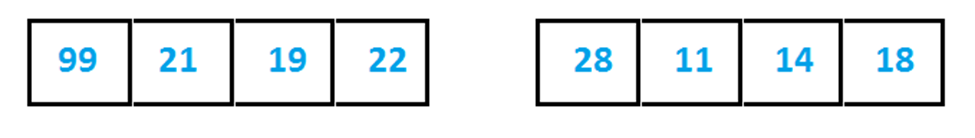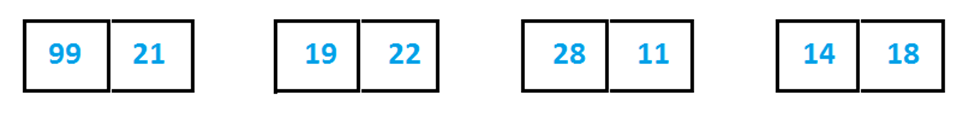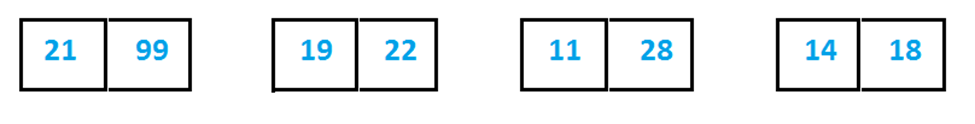Here you will learn about algorithm.

Merge sort is based on divide and conquer technique.

All we have to do is divide our array into 2 parts or sub-arrays and those sub-arrays will be divided into other two equal parts. We will divide the array in this manner until we get single element in each part because single element is already sorted.

After dividing the array into various sub-arrays having single element, now it is the to conquer or merge them together but in sorted manner.

## Python Merge Sort

### Example

Let’s see an example:

We have an array  [ 99, 21, 19, 22, 28, 11, , 18 ].There are 8 elements in the array. Break it into two equal parts.And repeat breaking until we get single element in each part or sub-array.We have single element in each sub-array. Now we have to merge them in the same way as had divided it, but in sorted manner.

Compare 99 with 21, 19 with 22, 28 with 11, 14 with 18 and place the smaller number first (for ascending order).here we have sorted arrays, keep conquering until we get complete sorted array.And finally our array is sorted.This was the theoretical concept, now take a look on how to implement this concept in programming.

First we’ll see an algorithm, in which we’ll divide given array into sub-arrays using recursion.

Second we’ll see algorithm for merging two sub-arrays into a single array but in sorted order.

### Algorithm

Mergesort(Array)

Step:1-  set n = length(Array)

If (n<2) then return

Step:2-  set mid = n/2

Initialize array “left” having size (mid)

Initialize array “right” having size (n-mid)

Step:3-  for i = 0 to mid – 1

left [i] = Array[i]

[exit loop]

for i = mid to n-1

right[i] = Array[i]

[exit loop]

Step:4-  call mergesort(left)

Call mergesort(right)

Call merge(left,right,Array)

merge(left, right, Array)

Step:1 – set i = 0, j =0,  k = 0

[i,j,k will represent the indexes of left,right and Array respectively. ]

Step:2 –   while (i<length(left) and j<length(right)

{

If (left[i] < right[j])

Then set Array[k] = left[i]

Increase i by 1

[end of if statement]

else

Set Array[k] = right[j]

Increase j  by 1

[end of else statement]

Increase  k by 1

[end of while loop]

Step:3- while (i < length(left))

{

Set Array[k] = left[i]

i = i + 1

k = k + 1

}

While (j < length(right))

{

Array[k] = Right[j]

Increase j by 1

Increase k by 1

}

### Program for Merge Sort in Python

Output

11 14 18 19 21 22 28 99

Comment below if you have doubts related to about python merge sort tutorial.